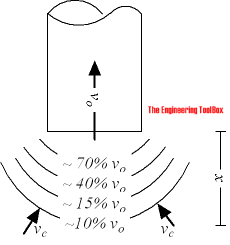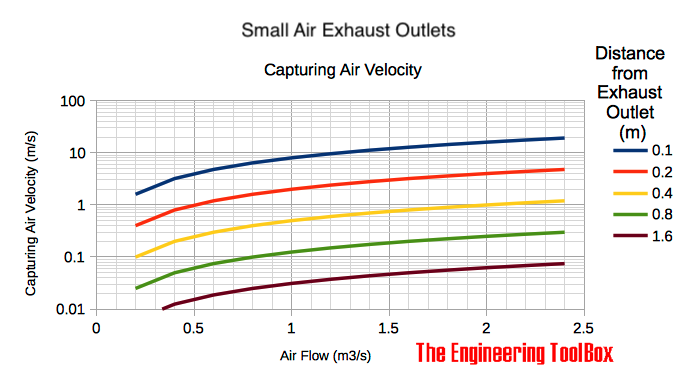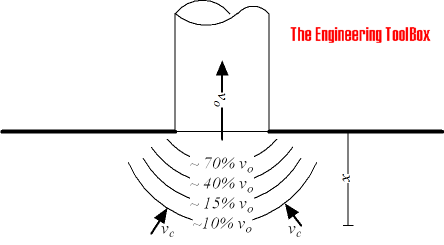Engineering ToolBox - Resources, Tools and Basic Information for Engineering and Design of Technical Applications!

# Exhaust Outlets - Capturing Air Velocities

## Capturing air velocity in front of an exhaust outlet - online exhaust outlet velocity calculator.The capturing air velocity in a distance x from an exhaust outlet can be calculated as

vc = q / (4 π x2)

= vo A / (12 x2)

= vo π d2 / (48 x2)                                    (1)

where

q = air volume flow (m3/s, ft3/s)

A = duct area (m2, ft2)

vc = capture air velocity in distance x from exhaust outlet (m/s, ft/s)

vo = air velocity in the exhaust outlet opening (m/s, ft/s)

d = diameter of exhaust outlet (m, ft)

x = distance from exhaust outlet (m, ft)

Note! According to line one of the equation (1) - the capture velocity is not dependent on the size of duct. This equation can only be used for exhaust outlets with relatively small diameters.For larger exhaust outlets equation (1) should be modified to

vc = vo A / (A + 10 x2)

= q / (A + 10 x2)                                      (2)

### Example - Capturing air velocity for a  smaller exhaust outlet

Capturing air velocity in distance 250 mm from a 250 mm duct with internal air velocity of 3 m/s can be calculated as

vc = (3 m/s) π (0.250 m)2 / (48 (0.250 m)2)

= 0.2 (m/s)

Note! The air velocity in distance one diameter from the duct outlet - is less than 10% of the duct air velocity.

### Exhaust Outlet Velocity Calculators

The velocity in distance x from an exhaust outlet can be calculated with the calculators below.

### Related Mobile Apps from The Engineering ToolBox- free apps for offline use on mobile devices

### Exhaust Outlets in Walls or with FlangesIf the exhaust outlet is in a wall or with a flange the evacuation efficiency is improved and can be expressed as

vc = 1.33 vo A / (A + 10 x2)

= 1.33 q / (A + 10 x2)                           (3)

The calculator above can be used if volume is multiplied with 1.33. Use of exhaust hoods improves the evacuation efficiency further.

## Related Topics

• ### Ventilation

Systems for ventilation and air handling - air change rates, ducts and pressure drops, charts and diagrams and more.

## Related Documents

• ### Duct Velocity

Calculate velocities in circular and rectangular ducts - imperial and SI-units - online calculator.
• ### Evacuation of Air - minimum Capture Velocities to avoid Pollution products transferred to the Room

Capture velocities to avoid pollution products from galvanic baths, spray painting boxes and more contaminating the surrounding room and environment.
• ### Exhaust Hoods

Sizing of exhaust hoods - air volume flow and capture velocities - online exhaust hood calculator.

## Search

Search is the most efficient way to navigate the Engineering ToolBox.

## Engineering ToolBox - SketchUp Extension - Online 3D modeling!

Add standard and customized parametric components - like flange beams, lumbers, piping, stairs and more - to your Sketchup model with the Engineering ToolBox - SketchUp Extension - enabled for use with older versions of the amazing SketchUp Make and the newer "up to date" SketchUp Pro . Add the Engineering ToolBox extension to your SketchUp Make/Pro from the Extension Warehouse !

We don't collect information from our users. More about

## Citation

• The Engineering ToolBox (2005). Exhaust Outlets - Capturing Air Velocities. [online] Available at: https://www.engineeringtoolbox.com/exhaust-outlet-d_1008.html [Accessed Day Month Year].

Modify the access date according your visit.

12.1.10